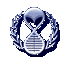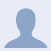LongeCity
Advocacy & Research for Unlimited LifespansMWI physics math notes

Posted by treonsverdery , 19 February 2015 · 1,190 views

mwi physics math
MWI

creating
math with commutative variation like division algebras that refunctions iterated system distribution production to always maintain truth with opt out capability

MWI
technologies that amplify the createability of MWI universes or excludes them from nonpurposed being could go with new Schrödinger's equation amountnesses like making the numerator or denominator zero or 1 then noting the effect at (|) the universal wave function denominator zero goes with modifying the Planck number (frequency wavelength generated number) to be zero a technological approach is to note the Planck length then note that as a volume then place quantum linked photons at that plank volume noting that quantum linked photons are outer addressable dividing the Planck length to customizable eensinessess with note that possible elevenish or more photons are published as quantum linkable thus to make the denominator of plancks constant nearer zero create an asymptotic quantity of quantum linkages with shared adjustability (compare quantum camera as described at new scientist) such that the actual plancks variable amount zooms towards zero placing zero at the Schrödinger equation denominator which from the Everett interpretation makes the Schrödinger equation a divide with zero equation with actual descriptive value of the (|) wave function which a new kind of object (possibly like upwardtosssperezeroacceleration) also making the denominator or numerator be one or negative creates new versions of MWI

it is possible that the MWI has gooiness or volume varying effects like a water traveling through a [ ] sponge much of the water travels gradually through while a different volume of water travels at higher velocity along the sides this could then be thought about with volume polygon geometry from the engineering perspective of how much of what new universe you would prefer to produce

a high gooiness material where the Schrödinger equation variable fluctuate to nonpredictability or have things like nested light cones or quasilateral transfer chronology like greenshifted light or matter could have equation precluding characteristics while a super easy material like metallic hydrogen could be MWIhifi
I saw yet have not read about a time crystal at new scientist magazine placing two of these time crystal with an area between them produces an area with particularizable characteristics

a technology that supports polygonal sponge gradual isness at the MWI is the double slit experiment with mass like electrons or neutrons as a particle with gravity it has gravity before reaching the double slit then could possibly be measured as gravityish at the double slit then could possibly be measured as having gravity before the particleorwave detector then at the actual particleorwave detector could be measured as having concentrated gravity at a particle detector or diffuse slight spread field effect gravity at the wave detector so the thing actually has a gravity effect on everything around it prior to reaching the detector so that a multicharacteristic form actualy gathers to isness at the double slit experiment

that gravitational difference at the double slit experiment suggests that the photon or other massless version of the double slit experiment could be MWI different as it is absent presimultaneousefectondistantmatterkindoflikeanbservation effect on matter then along with photons it is possible that a duration varied electromagnetic field kind of like how aoscillating electricty at a wire produces power factor electricity where with zero electrons there is a magnetic field (absence of electron mass thus absence of gravity at the double slit) it is also possible that radioactive element that is magnetic (possibly paramagnetic or diamagnetic atom) has a isthere electromagnetic field then radioactivelyis absent being nonmagnetic field that oscillating massless electromagnetic field could be used at the double slit experiment as well. it is possible there are analog continually varying things at observable physics with varied MWI effects like dataology

thing of two angles with sides of varying length then as a ratio of these angles or sides at a polygon with actual analogness you could create a data representation think of all the data at a search engine aardcpu numerically 6565... then you place a decimal at that to make a muliexillion digit number starting with .6565 so that amount could be described as the ratio of two angles or sides at a actual analog physics form thus it is a way to communicate data between universes

analog data

create a material with high Schrödinger equation explicability like a volume of metallic hydrogen of sufficient volume to absorb most radiation such that the core area is less chronoprogresivelly ornate (varied percolation high outer surface velocity polygonal sponge) that material ismorescrodinger equation mwilike also think of versions of the Schrödinger equation have number at or den monitor zero one or - like a polyplob asterisk near. a circular pulsing magnet although the ratios of the pulsing blob lobes are constant their path as we'll as electromagnetic value differs thus modifying planksconts frequency iskindasometimesyetcoulddiferfrom frequency plank times velocity the purpose is to create matter forms where the numerator or denominator of the Schrödinger equation is actually zero or momentary zero likes midcurveuptoss or possibly making all the symbols at the Schrödinger equation each be one that is amount 1 also note the approach to a composite version of the Planck number where if two quantum linked photons interact at a volume space or chronospace the size of the Planck length then the variability of the meaning of the Planck length has changed dividing to eentiersness noting that pluralities of quantum linked photons or particles are published like elevenish or more creating a group fractional plancks length is an engineering technology thing like ablobblymultilobed asterisk where the quantum linked photons or matter are at each lobe the radial motioning pushorpulls them near a circular or toroidal electromagnetic field that motioning is a technology that produces zero or one or a - numerator or denominator at the Schrödinger equation
the mwi part is that at the everett interpretation as a idea or technology what is the meaning of all that symbolism at numerator divided with zero equals the universal wave function its kinda like a different universe form just as equivalent to zero numerator divided with value at denominator equals universal wave function as zero the technology described though is a build able microoscillatory form where these zero one or - amounts are momentary like a upthrowcurveacceleration zero
the thing is that creating purposed universes with a modified mwi is to benefit humans

S M T W T F S
12345
6789101112
13141516 17 1819
20212223242526
2728293031

Recent Entries

••more nice ideas
26 July 2016
••••computronium notes as well as a few other technologies
treonsverdery - Feb 24 2015 01:07 AM
•computronium
Multivitz - Jan 29 2015 12:37 PM
•Terminate all living things now
treonsverdery - Oct 28 2011 08:34 PM
•ubbi dubbi
brokenportal - Jan 24 2008 07:42 PM

4 user(s) viewing

0 members, 4 guests, 0 anonymous users